MATLAB Function Referenceeig

Find eigenvalues and eigenvectors

Syntax

• ```d = eig(A)
d = eig(A,B)
[V,D] = eig(A)
[V,D] = eig(A,'nobalance')
[V,D] = eig(A,B)
[V,D] = eig(A,B,`flag`)
```

Description

```d = eig(A) ``` returns a vector of the eigenvalues of matrix `A`.

```d = eig(A,B) ``` returns a vector containing the generalized eigenvalues, if `A` and `B` are square matrices.

 Note    If `S` is sparse and symmetric, you can use `d = eig(S)` to returns the eigenvalues of `S`. If `S` is sparse but not symmetric, or if you want to return the eigenvectors of `S`, use the function `eigs` instead of `eig`.

```[V,D] = eig(A) ``` produces matrices of eigenvalues (`D`) and eigenvectors (`V`) of matrix `A`, so that `A*V` `=` `V*D`. Matrix `D` is the canonical form of `A`--a diagonal matrix with `A`'s eigenvalues on the main diagonal. Matrix `V` is the modal matrix--its columns are the eigenvectors of `A`.

If `W` is a matrix such that `W'*A = D*W'`, the columns of `W` are the left eigenvectors of `A`. Use `[W,D] = eig(A.'); W = conj(W)` to compute the left eigenvectors.

```[V,D] = eig(A,'nobalance') ``` finds eigenvalues and eigenvectors without a preliminary balancing step. Ordinarily, balancing improves the conditioning of the input matrix, enabling more accurate computation of the eigenvectors and eigenvalues. However, if a matrix contains small elements that are really due to roundoff error, balancing may scale them up to make them as significant as the other elements of the original matrix, leading to incorrect eigenvectors. Use the `nobalance` option in this event. See the `balance` function for more details.

```[V,D] = eig(A,B) ``` produces a diagonal matrix `D` of generalized eigenvalues and a full matrix `V` whose columns are the corresponding eigenvectors so that `A*V` `=` `B*V*D`.

```[V,D] = eig(A,B,flag) ``` specifies the algorithm used to compute eigenvalues and eigenvectors. `flag` can be:

 `'chol'` Computes the generalized eigenvalues of `A` and `B` using the Cholesky factorization of `B`. This is the default for symmetric (Hermitian) `A` and symmetric (Hermitian) positive definite `B`. `'qz'` Ignores the symmetry, if any, and uses the QZ algorithm as it would for nonsymmetric (non-Hermitian) `A` and `B`.

 Note    For `eig(A)`, the eigenvectors are scaled so that the norm of each is 1.0. For `eig(A,B)`, `eig(A,'nobalance')`, and `eig(A,B,flag)`, the eigenvectors are not normalized.

Remarks

The eigenvalue problem is to determine the nontrivial solutions of the equation

•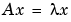where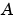is an `n`-by-`n` matrix,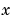is a length `n` column vector, and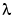is a scalar. The `n` values ofthat satisfy the equation are the eigenvalues, and the corresponding values ofare the right eigenvectors. In MATLAB, the function `eig` solves for the eigenvalues, and optionally the eigenvectors.

The generalized eigenvalue problem is to determine the nontrivial solutions of the equation

•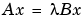where bothand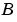are `n`-by-`n` matrices andis a scalar. The values ofthat satisfy the equation are the generalized eigenvalues and the corresponding values ofare the generalized right eigenvectors.

Ifis nonsingular, the problem could be solved by reducing it to a standard eigenvalue problem

•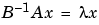Becausecan be singular, an alternative algorithm, called the QZ method, is necessary.

When a matrix has no repeated eigenvalues, the eigenvectors are always independent and the eigenvector matrix `V` diagonalizes the original matrix `A` if applied as a similarity transformation. However, if a matrix has repeated eigenvalues, it is not similar to a diagonal matrix unless it has a full (independent) set of eigenvectors. If the eigenvectors are not independent then the original matrix is said to be defective. Even if a matrix is defective, the solution from `eig` satisfies `A*X` `=` `X*D`.

Examples

The matrix

• ``````B = [ 3     -2      -.9    2*eps
``````     -2      4       1    -eps
``````     -eps/4  eps/2  -1     0
``````     -.5    -.5      .1    1   ];
``````

has elements on the order of roundoff error. It is an example for which the `nobalance` option is necessary to compute the eigenvectors correctly. Try the statements

• ```[VB,DB] = eig(B)
B*VB - VB*DB
[VN,DN] = eig(B,'nobalance')
B*VN - VN*DN
```

Algorithm

Inputs of Type Double

For inputs of type `double`, MATLAB uses the following LAPACK routines to compute eigenvalues and eigenvectors.

 Case Routine Real symmetric `A` `DSYEV` Real nonsymmetric `A:` With preliminary balance step `DGEEV` (with the scaling factor `SCLFAC` = `2` in `DGEBAL`, instead of the LAPACK default value of `8`) `d = eig(A,'nobalance')` `DGEHRD`, `DHSEQR` `[V,D] = eig(A,'nobalance')` `DGEHRD`, `DORGHR`, `DHSEQR`, `DTREVC` Hermitian `A` `ZHEEV` Non-Hermitian `A:` With preliminary balance step `ZGEEV` (with `SCLFAC = 2` instead of `8` in `ZGEBAL`) `d = eig(A,'nobalance')` `ZGEHRD`, `ZHSEQR` `[V,D] = eig(A,'nobalance')` `ZGEHRD`, `ZUNGHR`, `ZHSEQR`, `ZTREVC` Real symmetric `A`, symmetric positive definite `B`. `DSYGV` Special case:    `eig(A,B,'qz')` for real `A`, `B `    (same as real nonsymmetric `A`, real    general `B`) `DGGEV` Real nonsymmetric `A`, real general `B` `DGGEV` Complex Hermitian `A,`Hermitian positive definite `B`. `ZHEGV` Special case:     `eig(A,B,'qz')` for complex `A` or `B`    (same as complex non-Hermitian `A,`    complex `B`) `ZGGEV` Complex non-Hermitian `A,` complex `B` `ZGGEV`

Inputs of Type Single

For inputs of type `single`, MATLAB uses the following LAPACK routines to compute eigenvalues and eigenvectors.

 Case Routine Real symmetric `A` `SSYEV` Real nonsymmetric `A:` With preliminary balance step `SGEEV` (with the scaling factor `SCLFAC` = `2` in `SGEBAL`, instead of the LAPACK default value of `8`) `d = eig(A,'nobalance')` `SGEHRD`, `SHSEQR` `[V,D] = eig(A,'nobalance')` `SGEHRD`, `SORGHR`, `SHSEQR`, `STREVC` Hermitian `A` `CHEEV` Non-Hermitian `A:` With preliminary balance step `CGEEV` `d = eig(A,'nobalance')` `CGEHRD`, `CHSEQR` `[V,D] = eig(A,'nobalance')` `CGEHRD`, `CUNGHR`, `CHSEQR`, `CTREVC` Real symmetric `A`, symmetric positive definite `B`. `CSYGV` Special case:    `eig(A,B,'qz')` for real `A`, `B `    (same as real nonsymmetric `A`, real    general `B`) `SGGEV` Real nonsymmetric `A`, real general `B` `SGGEV` Complex Hermitian `A,`Hermitian positive definite `B`. `CHEGV` Special case:     `eig(A,B,'qz')` for complex `A` or `B`    (same as complex non-Hermitian `A,`    complex `B`) `CGGEV` Complex non-Hermitian `A,` complex `B` `CGGEV`

`balance`, `condeig`, `eigs`, `hess`, `qz`, `schur`